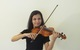•(Edited )

•I ended up getting it work in Visual Studio Code by adding a function to add up the array.  I've updated the link in above post to the test form.  I've tried adding it as custom script in a message but no luck.  Any ideas?  Maybe you have a built in function that will add up the array i can use?

•Hi Mike,

Sorry, I didn't understand your question. Can you explain in some detail what you are trying to achieve in this form?

-Prajakta

•This is working in Visual Studio Code.  Money103 is checkbox values (from a repeat), Money97 is the sum of those values chosen from checkbox's.  Using reduce with the function getsum or reduce with ((a, b) => a + b, 0); gets the results i want, but not having any luck getting it to work in frevvo.  I've tried both ways and still get the checkbox results only in Money97.  I need to be able to get the value of the arrays (which will be dynamically set in actual form, just using this as a test case).  The form in google drive is my test case and I have tried to add the function in a message control and use the inline code as well.  I tried to do this with visual rules builder, but it sees the values of the checkboxes as strings and gives me an error that they are not numbers.

//var Money103=[[100,200,300]];
var Money103=[[100,200,300],[200,300]];
var sum_result, sum_total;
var Money97=[];
function getSum(total, num) {
}

for (let i = 0; i < Money103.length; i++) {
if (Boolean(Money103[i])) {

//This
Money97[i] = Money103[i].reduce((a, b) => a + b, 0);
//Or this
Money97[i] = Money103[i].reduce(getSum);

}console.log(Money97[i]);
}

•Ok, now I get it. Try this business rule in your form:

 ```function getSum(total, num) { return total + num; } var event = form.load || Repeat99.itemRemoved || Repeat99.itemAdded; var tot = 0; for (let i = 0; i < Money103.value.length; i++) { if (Boolean(Money103[i].value)) { Money97[i].value = (Money103[i].value.map(Number)).reduce(getSum); } } ```

The arr.map(Number) method runs over all items in array, executes the Number function on every item and adds the result into a new array.

Let me know if that helps.

•That did it :).  I was so close, but so far...  You rock